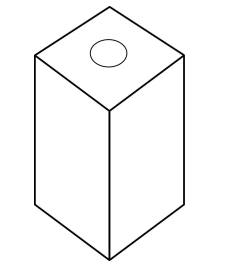# IMAT 2016 Q3 [Painted Tins]

Below is a picture of a biscuit tin with four identical sides. A set of these tins is to be made that are all distinguishable from each other by colour alone. The manufacturer will paint each of the four sides either red or blue. The top and bottom are not painted.What is the greatest number of different tins that can be made?

A. 6
B. 7
C. 8
D. 4
E. 5

1 Like

Simple steps to solve word problems:

• Underline key information
• Determine what they are trying to ask, and what you will need to solve it
• Eliminate any non-essential information
• Draw a picture, graph, or equation
• In moments of high stress like exam taking, always work with the paper they give you to avoid careless mistakes.
• Solve.

Approach: find all the possible combinations of red and blue, while considering that the tin could be all red or all blue. Also, note that some combinations will be the same depending on orientation.

Let ‘R’ stand for red side, and ‘B’ stand for blue side.

1. B B B B

2. R R R R

3. R R B B

4. B R B R

5. B B B R

6. R R R B

Anything else?

What about R B B R? No, this is the same as R R B B. Remember this is a tin with 4 sides.

Since there are no other possible combinations, 6 is the max possible.

\fcolorbox{red}{grey!30}{Therefore the answer is A, \$6\$.}
1 Like

Hi, the answer key suggests B, 7, I’m wondering what the 7th combination could be.

hello!
i found 7 and the answer key i checked was 6i believe different answers keys are around so better if you just use the solution only

Oh I see… Thank you so much!

1 Like

not sure what you mean because this answer key says 2016 Q3 (this question) is A!

1 Like